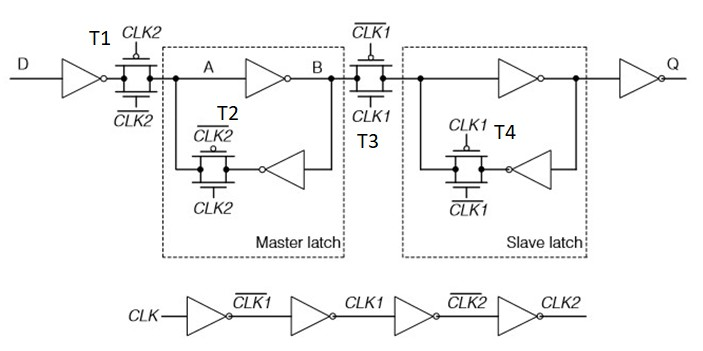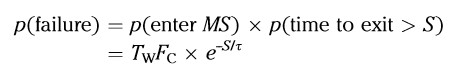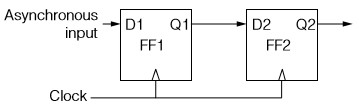Make it to the Right and Larger Audience

Blog

Here is a good tutorial of flip flop metastability and clock synchronization:

Metastability and Synchronization

Take-aways from above tutorial:

How FF works?

Most of time and most of us rtl designers treat flipflop as black box. Given clock and data, as long as setup and hold are met, output should be there others ‘x’ in sim and random out in field. However, some time we need to look inside.

Below is a typical master slave positive-edge FF. When CLK is 0, transmission gate pair T1 is on, T2 is off, T3 is off, and T4 is on. So node A and B follows data in D and output Q is not affected by D and still outputs FF existing state. When CLK is 1, T1 is off, T2 is on, T3 is on, and T4 is off. So A-B loop keeps D state and Q reflects D state too.When metastability happens?

When clock is 0, node A and B changes with D. If clock goes to 1 while A and B doesn’t reach full VDD or 0, metastability happens.

Hong long does it take for FF to go out of metastable state?

The tutorial has derivation. In short, the time FF needs to be out of MS is not a constant number. It is random governed by a known probability. Let’s say we give S for FF to recover, the failure probability (FF is still in MS after S elapses) iswhere$\tau$ is a timing constant related to chip process. Tw is setup window. Fc is FF clock rate.

The mean time between failures (MTBF) isWhere$F_D$ is data pin change rate.

Tutorial gives an example. For 28nm process,$\tau$ =10ps, Tw=20ps. Use Fc=1Ghz and let S=Tc, allowing one clock cycle to recover, MTBF=4×10^29 years. Very safe.

So we add two FF synchronizer as below.

Why these two FFs need to be placed close to each other?In above calculation, we let S=Tc so allow one clock cycle to recover. If synchronizer FFs are not close to each other and routing introduces some delay,  Then we only give S=Tc-delay for FF to recover so MTBF suffers.

As the tutorial said, “the bottom line is that Q2 is never metastable (except, maybe, once every MTBF years)”. We can’t use Q1 to drive logic since gate delay will eat some recovery time.

What is the voltage and temperature effect?

The tutorial gives an example. With the same 28nm process but in the case of low voltage and high temperature,$\tau$ =100ps, Tw=200ps. Still use Fc=1Ghz and S=Tc, MTBF is just about one minute.

Tutorial said use four flip-flops and S becomes 3Tc and MTBF jumps to 1,000 years. To make S=3Tc, looks four flip-flops are not needed and just don’t sample Q1 until 3Tc passes.

There are more information in the tutorial, especially on the handshaking synchronization.Author brief is empty
Groups:

Tags:

## clocksynchronization

1.0
-0

How big MTBF is good enough? The example given has such a big MTBF that even 10th and 100th of it is big. So we can just use one ff to sync assuming extra logic delay is small as 10th or 100th?

4
2.Arcis 3 years ago
0
-0

So for slow clk, can use 1st ff out directly. It is also process dependant. Each process needs to calculate fail rate to chech 2 ff synchronizer works or not

0
3.Arcis 3 years ago
0
-0

To the point digest.

4